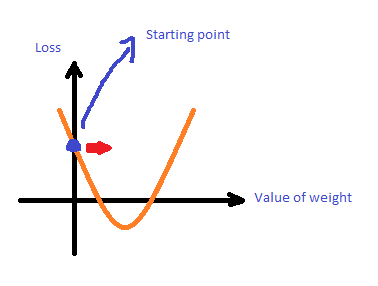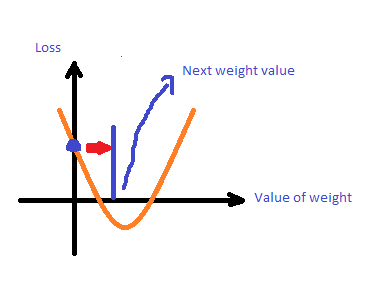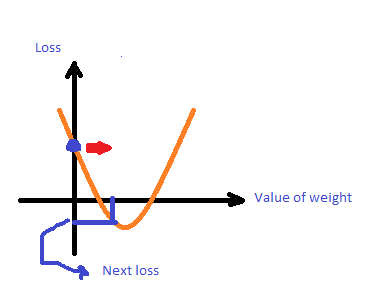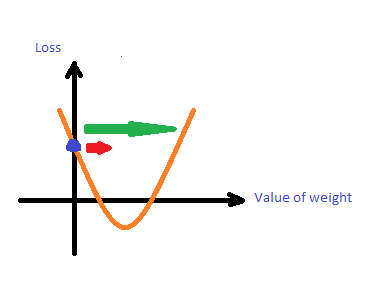2019 iT 邦幫忙鐵人賽DAY 6
0
AI & Data

[Day 6] ML中的reducing loss

Ref.: Reducing Loss

An Iterative ApproachSquared loss = (y - y')^2 = (y - 0)^2 = y^2Gradient f': prediction function 偏微分 (f')，(loss, weight) 的點在該函數對應的方向有最大的增加量
Gradient * -1 -f': prediction function 偏微分 (f')後乘以-1，(loss, weight) 的點在該函數對應的方向有最大的減少量Learning Rate (step size)w = w - LearningRate * gradient(w)